# Course 18.086: Mathematical Methods for Engineers II

Spring 2006: Room 3-370, MWF1

###Department of MathematicsMassachusetts Institute of Technology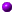MESSAGE TO THE CLASS   I have read the projects and I am very satisfied -- I think you will be satisfied with course grades. If you don't need the copy I have, I will keep it. (Hongmei can pick hers up except pages 1-2.) I wrote small notes on the cover pages and Brett will have those any afternoon in 2-235. THANK YOU for taking the course.   Gilbert Strang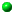Link to paper on "Approximate and Incomplete Factorizations"   http://www.library.uu.nl/digiarchief/dip/dispute/2001-0621-115821/proc.pdf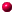Is there a simple way to count the fill-in in elimination ?

Run chol (or lu) and use "nnz" (number of non-zeros).

 >> % Generate discrete Laplacian operator
>> A=delsq(numgrid('S',100));
>> % Choleskey factorize
>> R=chol(A);
>> nnz(R)
ans =
941289

>> % As above, but permute rows & columns by approximate minimum degree
>> p=symamd(A);
>> R=chol(A(p,p));
>> nnz(R)
ans =
184917Does min degree just take nodes a row at a time (if it breaks ties correctly)   If that is N^3 -- and would sparse backslash do it ?? --

Yes it does, it is a "greedy algorithm." The approximate one might be a little different, but in principle it does the same thing. Sparse backslash does this automatically. If symmetric, then it uses "symmmd" (symmetric minimum degree), then "chol." If non-symmetric, "colamd" (column approximate minimum degree), then umfpack.this is about as fast as possible except for Fast Poisson Solvers ?

Well, it is probably as fast as possible for Gaussian elimination. But iterative solvers do better, I think CG with Incomplete Choleskey should be N^(2.5). Then of course fast solvers, FFT-based and multigrid.Homework for Monday April 11 (start now please!!) from text pages 403-411 and also Section 3.6 of the "new book". Answer and turn in problems 1,2,3 in that Section 3.6

Develop a code for INCOMPLETE LU and try it on the 2D matrix for increasing N. You can make it a general code or specialize it to these particular matrices (and decide which entries to keep in the approximate factors L and U). Try on A2D u = random right side. You could use eig to find the spectral radius of the preconditioned matrix B. That matrix is I - inv(P)A2D where P ≈ LU. Of course if P = A2D then B = 0 and convergence in 1 step.Read sections 3.6 and 3.7 (later 7.2 and 7.4) from the new book   3.6   3.7   7.2   7.4Quiz on Friday March 11: Exact solutions and finite difference approximations to the equations discussed from Sections 6.4-6.6. Please bring printout of code and exact and approximate solutions at t=1 to the heat equation ut = uxx with u(x,0) = \delta(x). Choose any difference method and any stable \Delta t and \Delta x: grade does not depend on order of accuracy !M. Benzi, G. H. Golub and J. Liesen, "Numerical solution of saddle point problems", Acta Numerica, 14 (2005), pp 1-137 (to appear).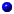Lecturer: Gilbert Strang, gs@math.mit.edu. Office: 2-240. Office hours: Mon 3-4, Wed 2-3.Graders: Zhou Zhang (zhangou@math.mit.edu) and Fangyun Yang (fangyun@math.mit.edu). Office: 2-251. Office hour: Tues 11:30-12:30.Textbook: Introduction to Applied Mathematics by Gilbert StrangCourse information:  (ps,pdf)Homework 1   Due IN CLASS WED 2/9 FRI 2/11 (stapled if possible)   PLEASE **PRINT YOUR NAME**   6.4: 1-5, 13-15, 22, 29;   6.5: 1-2, 17Homework 2   for FRIDAY FEB 25 MON FEB 28   6.5: 16 and 19 and (Find a stable difference method for ut = uxxx)   6.6: 1, 2, 5, 9   ALSO SOLVE ut + uux = 0 to t = 4 with u(x,0) = 1 and 0, also 0 and 1, as discussed in class (Lax-Friedrichs method).     Class MATLAB codes:   1   2   3   4   5No final.Exams: 2 or 3 mid-term exams. Open books and notes.Computational project.Homework: One problem set due in class each week, except exam weeks.Grading: Exams 50%, project 30%, homework 20%.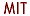Copyright © 2002 Massachusetts Institute of Technology